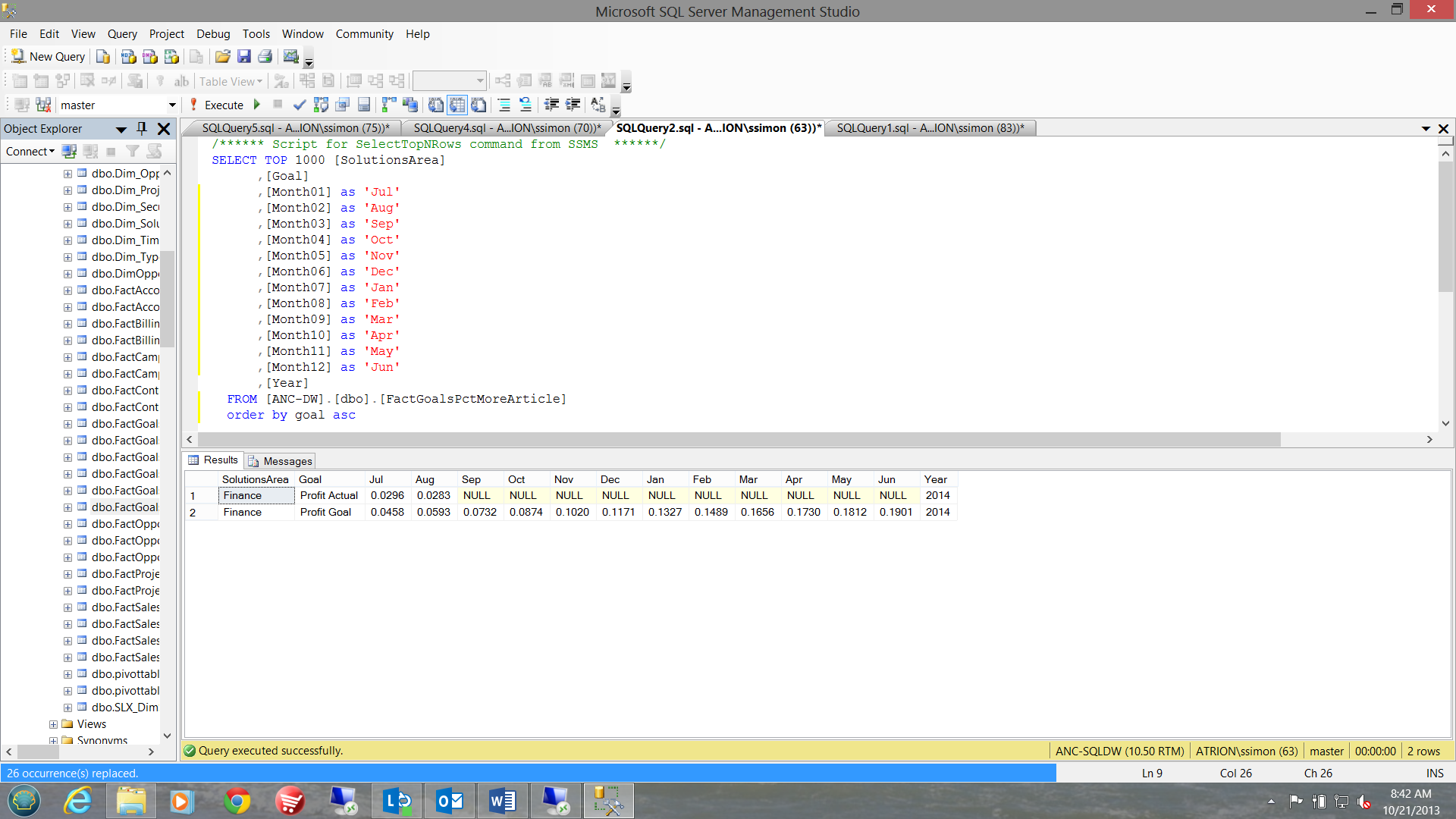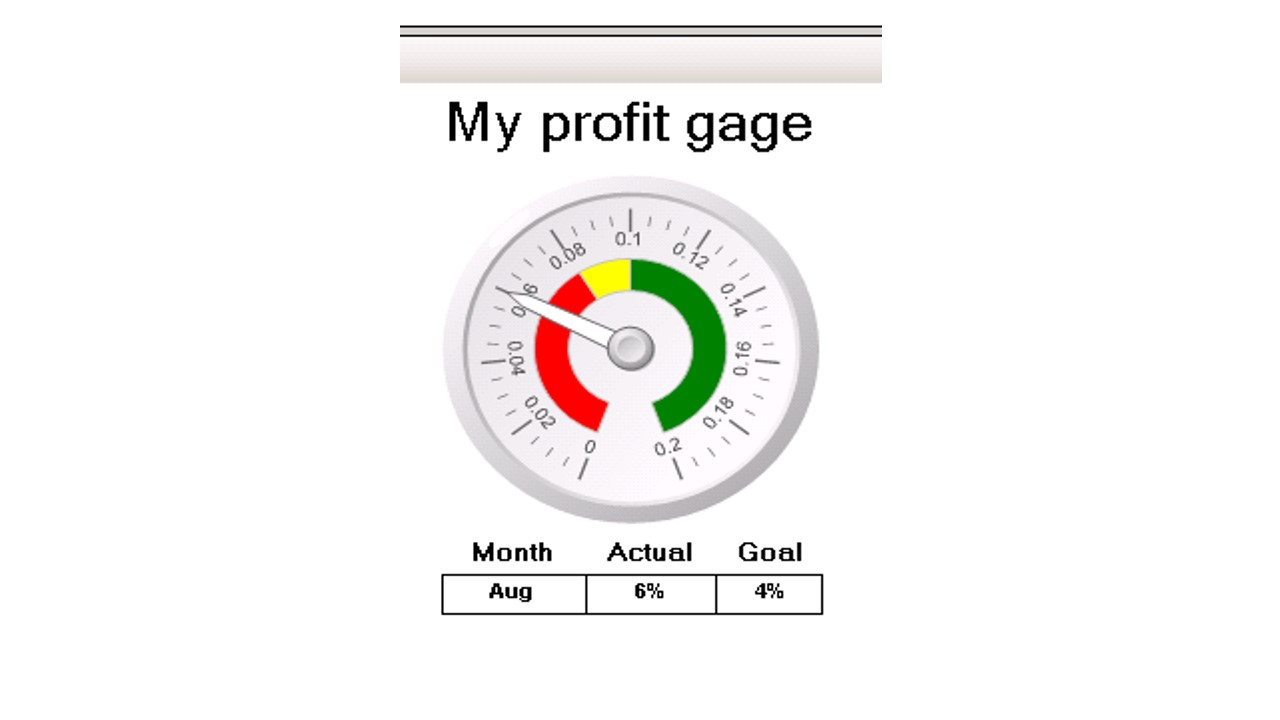# Monthly profit percentage techniques

,

Introduction

A few days ago, a friend of mine approached me with an interesting challenge. What he was trying to achieve was to extract monthly profit percentages from a SQL Server table (along with the projected monthly goal) and to place the latest month’s actuals into an SSRS gauge and additionally, the relevant data into a report matrix.

This lead to two interesting issues, the first that the profit calculations are 60 -90 days in arrears (thus DATEPART with Getdate() was not always accurate) and that the firm’s financial year starts on July 1 and end on June 30. No one can be certain as to when the monthly actual is available and the value may be amended at any time.

This is how I tacked the problem.

The raw data

As a starting point, Figure 1 shows the raw data within the table. The field names are actually MONTH01 through MONTH12. I have used month names in the screen dump below, purely for clarity.Fig 1

This screen dump was run in late October. The reader will note that actual figures are available for July and August only.  The main report contains a ‘gauge’, with its corresponding matrix. The matrix shows the latest month, along with the profit for that month and the corresponding goal. See Fig 2.Fig 2

We obtain the SSRS datasets via the following code snippet. Note the usage of table variables.
The same query is used for both the gauge and the matrix. The gauge works off of the ‘actual’ value within the result set.

Also note the query predicate in RED towards the bottom of the code.This is used to determine which month must appear within the matrix. Please remember that what we really want are the figures for most current month with NON NULL actual values.

use [ANC-DW]
go

create procedure speedomter
as
declare @sql as varchar(2000)
declare @actual table (sortkey int,actual decimal(10,3))
declare @goal   table (sortkey int,goal decimal(10,3))
declare @final   table (sortkey int,value decimal(10,3))
drop table #rawdata1
drop table #rawdata2
drop table #temp1

declare @kounter as int
set @kounter = 1
select Month01,month02,month03,month04,month05,month06,month07,month08
,month09,month10,month11,month12
into #rawdata1 from dbo.FactGoalsPctMoreArticle
where goal like '%actual%'

--set the goals

select Month01,month02,month03,month04,month05,month06,month07,month08
,month09,month10,month11,month12 into
#rawdata2 from dbo.FactGoalsPctMoreArticle
where goal like '%Goal%'
WHILE @kounter < 13
BEGIN
Insert into @actual (sortkey, actual)
select
case
when @kounter =1 then 1
when @kounter =2 then 2
when @kounter =3 then 3
when @kounter =4 then 4
when @kounter =5 then 5
when @kounter =6 then 6
when @kounter =7 then 7
when @kounter =8 then 8
when @kounter =9 then 9
when @kounter =10 then 10
when @kounter =11 then 11   else 12
end as sortkey,

case
when @kounter =1 then Month01
when @kounter =2 then Month02
when @kounter =3 then Month03
when @kounter =4 then month04
when @kounter =5 then Month05
when @kounter =6 then Month06
when @kounter =7 then Month07
when @kounter =8 then Month08
when @kounter =9 then Month09
when @kounter =10 then Month10
when @kounter =11 then Month11   else Month12
end as actual from #rawdata1

set @kounter = @kounter + 1
END

set @kounter=1

--Now that we have the actuals, let us obtain the goals

WHILE @kounter < 13
BEGIN
Insert into @goal (sortkey, goal)
select
case
when @kounter =1 then 1
when @kounter =2 then 2
when @kounter =3 then 3
when @kounter =4 then 4
when @kounter =5 then 5
when @kounter =6 then 6
when @kounter =7 then 7
when @kounter =8 then 8
when @kounter =9 then 9
when @kounter =10 then 10
when @kounter =11 then 11   else 12
end as sortkey,
case
when @kounter =1 then Month01
when @kounter =2 then Month02
when @kounter =3 then Month03
when @kounter =4 then month04
when @kounter =5 then Month05
when @kounter =6 then Month06
when @kounter =7 then Month07
when @kounter =8 then Month08
when @kounter =9 then Month09
when @kounter =10 then Month10
when @kounter =11 then Month11   else Month12
end as goal from #rawdata2

set @kounter = @kounter + 1

END

select a.sortkey, a.actual,b.goal into #temp1 from @actual a
inner join @goal b
on a.sortkey = b.sortkey
where not actual is null
order by sortkey desc

select top 1
case
when sortkey = 1 then 'Jul'
when sortkey = 2 then 'Aug'
when sortkey = 3 then 'Sep'
when sortkey = 4 then 'Oct'
when sortkey = 5 then 'Nov'
when sortkey = 6 then 'Dec'
when sortkey = 7 then 'Jan'
when sortkey = 8 then 'Feb'
when sortkey = 9 then 'Mar'
when sortkey = 10 then 'Apr'
when sortkey = 11 then 'May'
when sortkey = 12 then 'Jun'
end as Monthee, actual, goal from #temp1

## Rate

You rated this post out of 5. Change rating

## Rate

You rated this post out of 5. Change rating